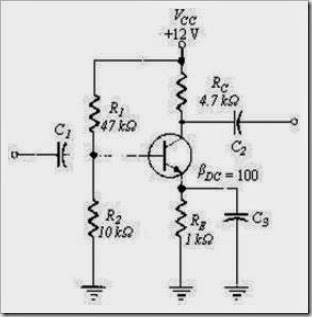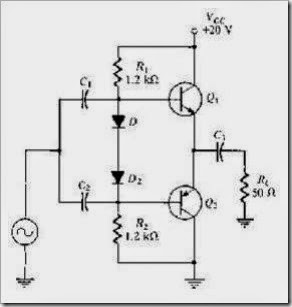# MCQs in Floyd: Power Amplifiers

(Last Updated On: December 8, 2017)

This is the Multiple Choice Questions in Power Amplifiers from the book Electronic Devices – Electron Flow Version and Conventional Current Version 8th Edition by Thomas L. Floyd. If you are looking for a reviewer in Electronics Engineering this will definitely help. I can assure you that this will be a great help in reviewing the book in preparation for your Board Exam. Make sure to familiarize each and every questions to increase the chance of passing the ECE Board Exam.

### Online Questions and Answers Topic Outline

• MCQs in Power Amplifiers
• MCQs in Class A Power Amplifier
• MCQs in Class A Power Amplifier
• MCQs in Class AB Push-Pull Amplifiers
• MCQs in Class C Power Amplifier

### Practice Exam Test Questions

Choose the letter of the best answer in each questions.

1. For BJT power transistors, the collector terminal is always connected to the transistor’s case
• A) for easy circuit connection.
• B) to prevent shorts.
• C) because the collector terminal is the critical terminal for heat dissipation.
• D) because the collector terminal is located nearest the case.

2. Quiescent power is the power dissipation of a transistor

• A) with no signal input.
• D) along the dc load line.

3. A class B amplifier operates in the linear region for

• A) slightly more than 180° of the input cycle.
• B) 360° of the input cycle.
• C) slightly less than 180° of the input cycle.
• D) much less than 180° of the input cycle.
4. In a class AB amplifier, if the VBE drops are not matched to the diode drops or if the diodes are not in thermal equilibrium with the transistors, this can result in
• A) a current mirror.
• B) diode separation.
• C) crossover distortion.
• D) thermal runaway.

5. Which amplifier is commonly used as a frequency multiplier?

• A) class A
• B) class B
• C) class C
• D) all of the above

6. The least efficient amplifier among all classes is

• A) class B.
• B) class A.
• C) class AB.
• D) class C.
7. A class A amplifier has a voltage gain of 30 and a current gain of 25. What is the power gain?
• A) 30
• B) 25
• C) 1.2
• D) 750
8. You have an application for a power amplifier to operate on FM radio frequencies. The most likely choice would be a _____ amplifier.
• A) class A
• B) class B
• C) class C
• D) class AB
9. A class A amplifier with RC = 3.3 kΩ and RE = 1.2 kΩ has a VCC = 20 V. Find IC(sat).
• A) 4.4 mA
• B) 6.1 mA
• C) 16.7 mA
• D) 20 mA

10. Refer to Figure 7-1. The dc voltage on the collector, VC, isFigure 7-1

• A) 5.4 V.
• B) 6.6 V.
• C) 12 V.
• D) 0 V.
11. Refer to Figure 9-1. This amplifier is operating as a _____ amplifier.
• A) class A
• B) class B
• C) class AB
• D) class C
12. Refer to Figure 7-2. This amplifier is operating as a _____ amplifier.
• A) class A
• B) class B
• C) class AB
• D) class C
13. Refer to Figure 7-2. The approximate voltages on the base, collector, and emitter, respectively, are
• A) 0.7 V, 6.8 V, 0 V.
• B) 0 V, 0 V, 0 V.
• C) 0.7 V, 15 V, 0 V.
• D) 0.7 V, 0 V, 15 V.

14. Refer to Figure 7-2. The maximum efficiency of this amplifier is

15. Refer to Figure 7-3. Determine VB1.Figure 7-3

• A) 0 V
• B) 0.7 V
• C) 9.3 V
• D) 10.7 V

16. Refer to Figure 7-3. Calculate VB2.

• A) 0 V
• B) 0.7 V
• C) 9.3 V
• D) 10.7 V
17. Refer to Figure 7-3. You have an oscilloscope across RL and it shows a zero signal voltage. The problem might be that
• A) C3 is open.
• B) BE1 is open.
• C) BE2 is open.
• D) R1 is open.
18. Refer to Figure 7-3. You find that this amplifier only shows the negative alternation at the output. The possible trouble might be that
• A) C3 is shorted.
• B) BE1 is open.
• C) BE2 is open.
• D) R1 is open.
19. Refer to Figure 7-3. You find that there is no output signal. You measure the dc voltage of Q1 emitter and find it equal to 0 V. The trouble might be
• A) D1 is shorted.
• B) D2 is shorted.
• C) R1 is open.
• D) no trouble, everything is normal.
20. Refer to Figure 7-3. You find that there is an input signal on the base of Q1 and Q2. However, there is no output signal. You then measure the dc voltages on Q2 and find them to be all 0 V. The possible trouble might be
• A) C3 is shorted.
• B) C1 is open.
• C) RL is shorted.
• D) VCC is 0 V.
21. A class C amplifier has a tank circuit in the output. The amplifier is conducting only 28°. The output voltage is
• A) 0 V.
• B) a dc value equal to VCC.
• C) a sine wave.
• D) a square wave with a frequency determined by the tank.
22. In practice, the efficiency of a capacitively coupled class A amplifier is about _____%.
• A) 25
• B) 40
• C) 70
• D) 10
23. The Q-point is at cutoff for class _____ operation.
• A) A
• B) B
• C) C
• D) AB
24. Class _____ amplifiers are normally operated in a push-pull configuration in order to produce an output that is a replica of the input.
• A) A
• B) B
• C) C
• D) AB

25. The maximum efficiency of a class B amplifier is _____ percent.

• A) 50
• B) 25
• C) 70
• D) 79
26. A class _____ amplifier is biased slightly above cutoff and operates in the linear region for slightly more than 180º of the input cycle.
• A) A
• B) B
• C) C
• D) AB
27. Which class of amplifier operates in the linear region for only a small part of the input cycle?
• A) A
• B) B
• C) C
• D) AB

28. The principal advantage(s) of MOSFETs over BJTs is (are)

• A) their biasing networks are simpler.
• B) their drive requirements are simpler.
• C) they can be connected in parallel for added drive capability.
• D) all of the above

29. The principal advantage(s) of BJTs over MOSFETs is (are) that

• A) voltage drop across the transistor is important.
• B) they are not as prone to ESD.
• C) both of the above
• D) none of the above

30. The class _____ amplifier is biased below cutoff.

• A) A
• B) AB
• C) B
• D) C